Courses
Courses for Kids
Free study material
Free LIVE classes
More

# Subtraction Year 3 - Learn Subtraction with ExamplesLIVE
Join Vedantu’s FREE Mastercalss

## Before that let us briefly revise subtraction - Subtraction has three parts, subtrahend, minuend, and the difference. Subtrahend and minuend remain at the left side of the equals to sign while the difference remains at the right of the equal to sign.

Now, we learn the concept of subtraction without and with borrowing using 4-digit or 5-digit numbers along with some solved examples. Also, we will understand how borrowing takes place while subtracting numbers.

## Subtraction without Borrowing for Class 3 Students

The same 3-digit numbers subtraction method is applicable to 4-digits, 5-digits, and 6-digits numbers.

Now, let us learn 4-digits or 5-digits subtraction without borrowing. A few examples are shown below:

Example 1. Subtract 6027 from 7649 and determine the result.

Solution: First, place the digits of the numbers according to place value in column format as shown in the image.

Step 1 - Subtract the digits under ones place as shown below: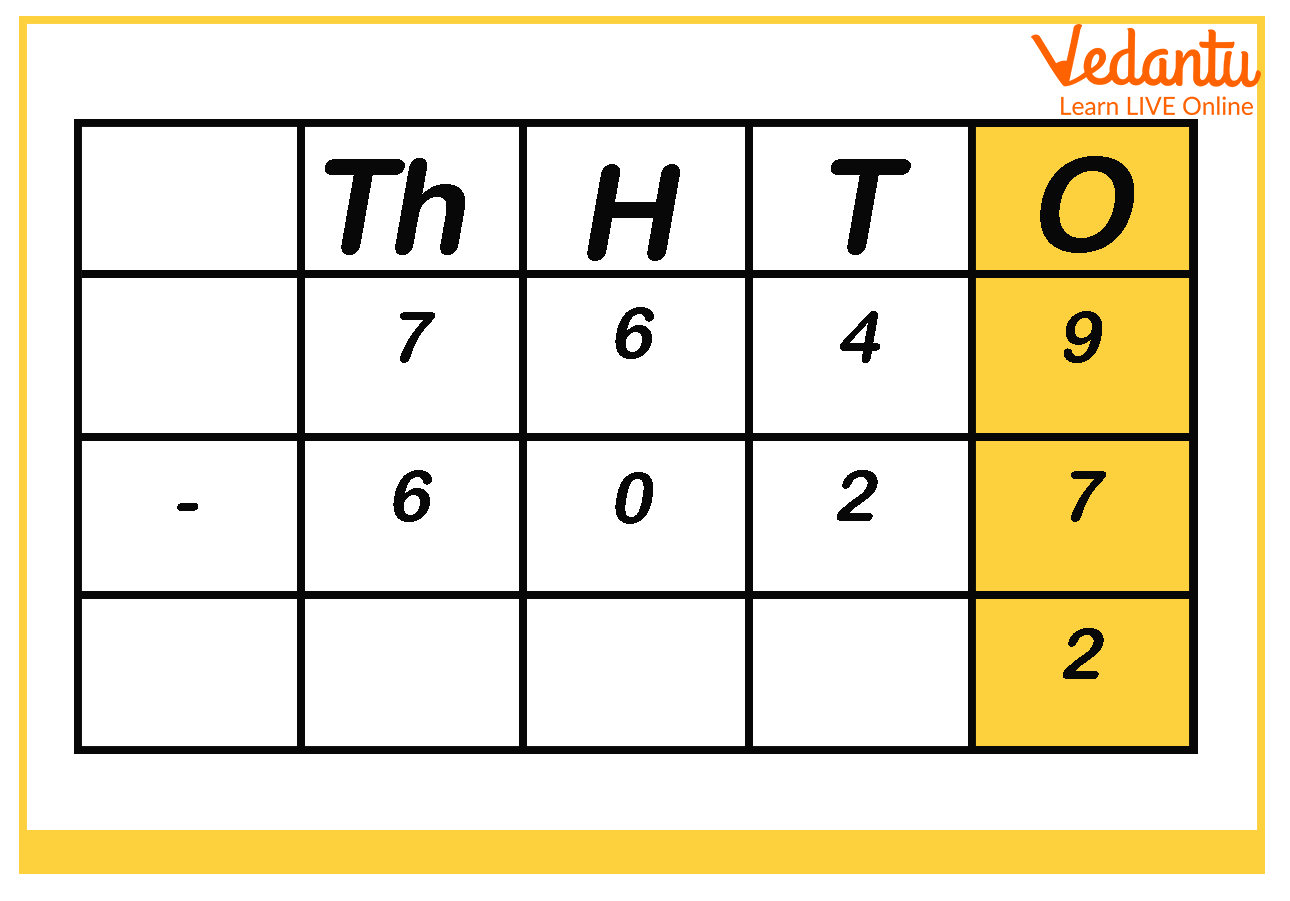Step 2: Then, subtract the digits under tens place as you can see in the picture below.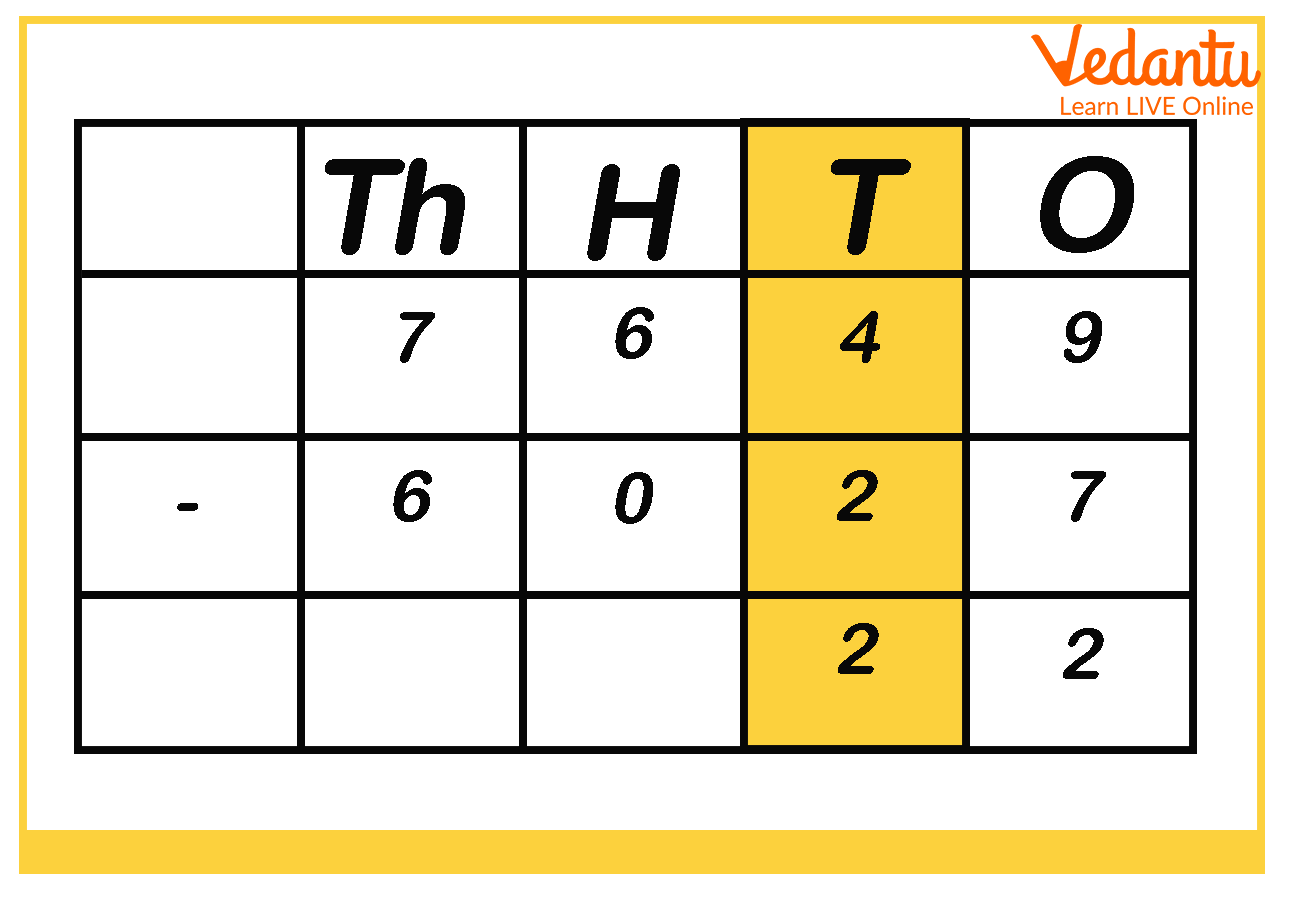Step 3: Now, subtract the digits under hundreds place.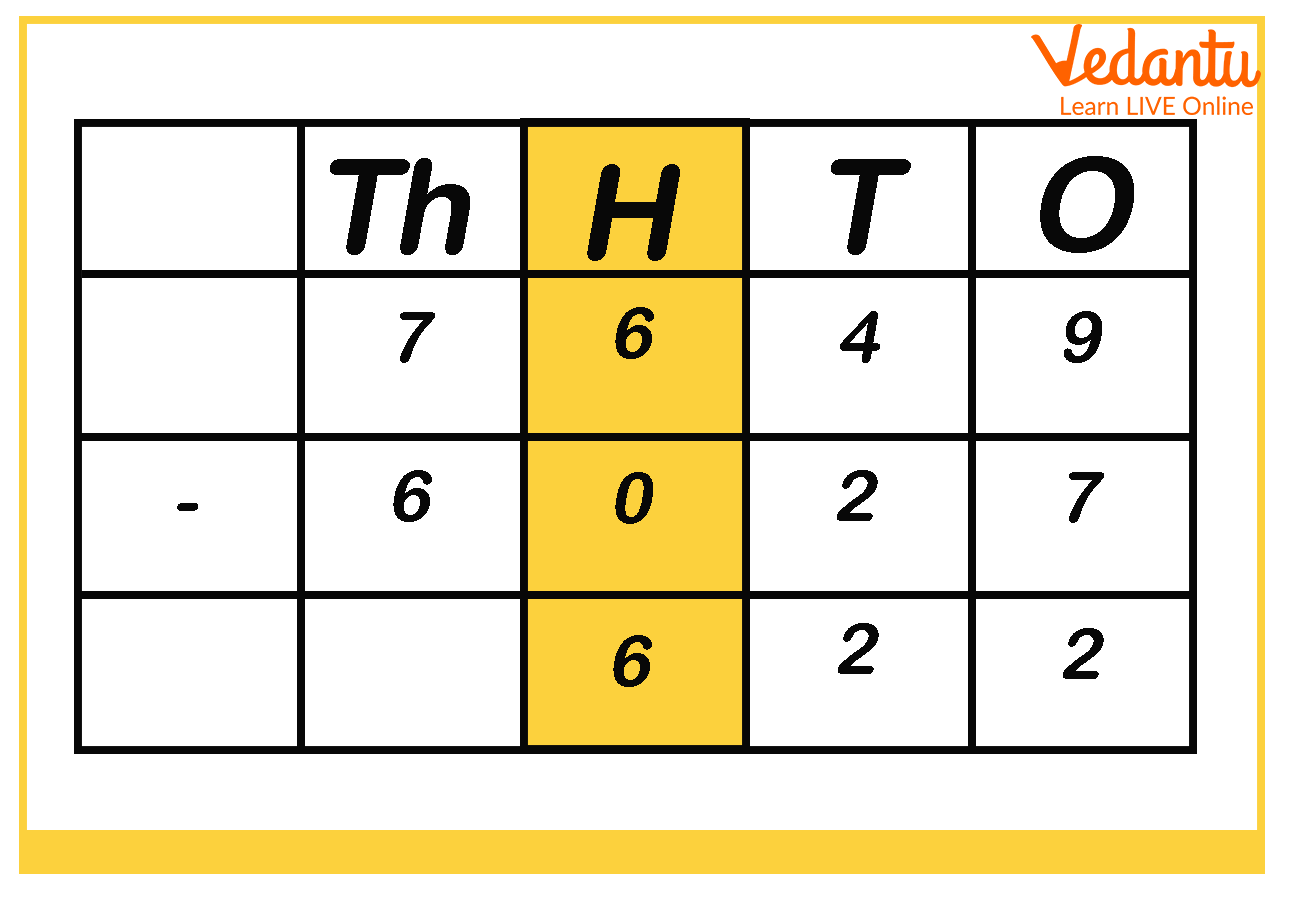Step 4: Finally, subtract the digits under thousands place.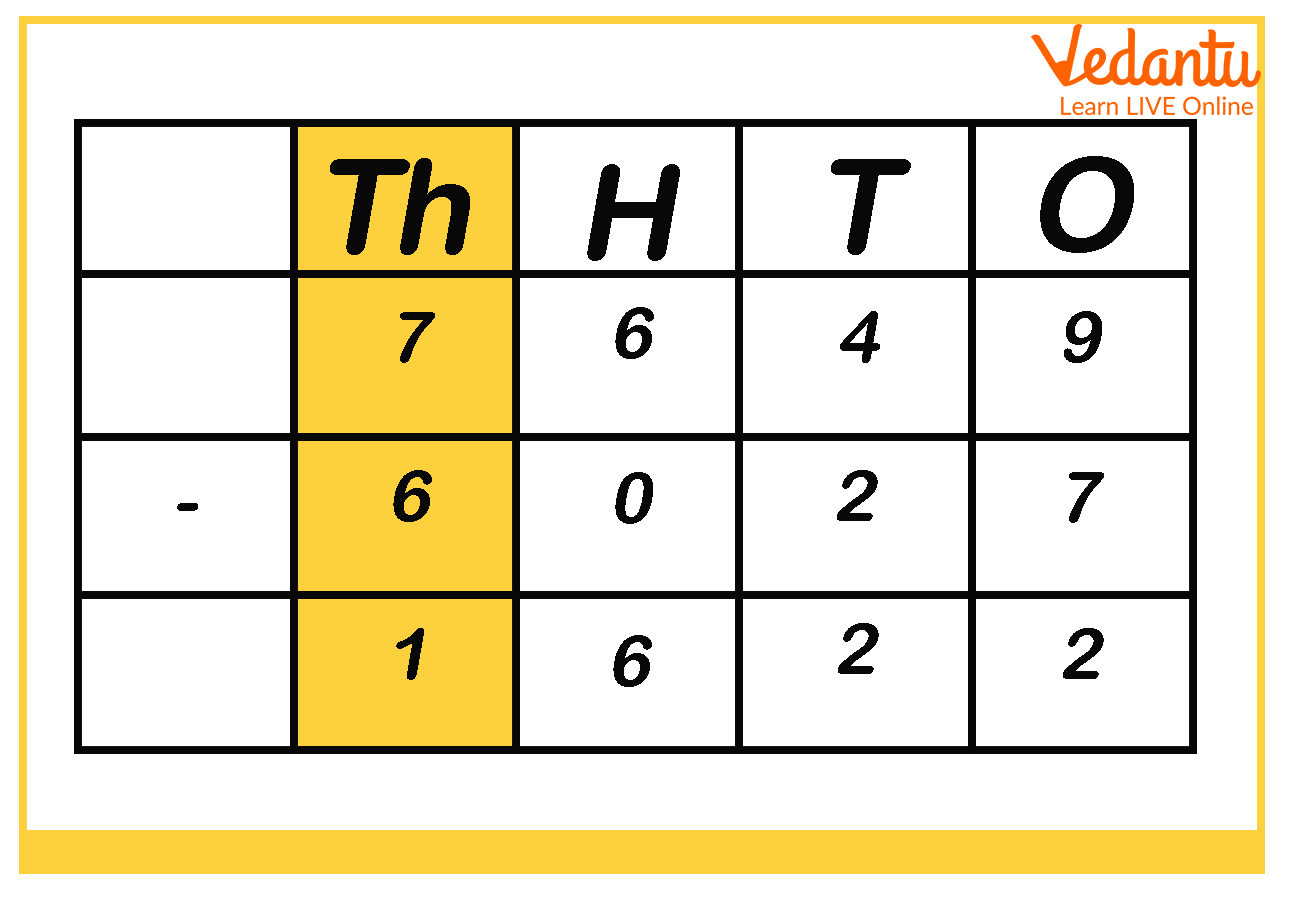So, from the last image, we get 1622 as the result, which means subtracting 6027 from 7649 we get 1622 as the answer.

Now, let us have a look at an example of subtraction with borrowing.

### Subtraction with Borrowing for Class 3 Students

We are already well-versed with borrowing on 2 and 3-digit numbers. Now, we will use the same method for subtraction of 4 and 5-digit numbers. A few examples are given below.

Example 1. Subtract 29627 from 76322.

Solution: Place the digits of the numbers according to the place value in column format as shown in the image below.

Step 1 - Subtract the digits under ones place as shown below: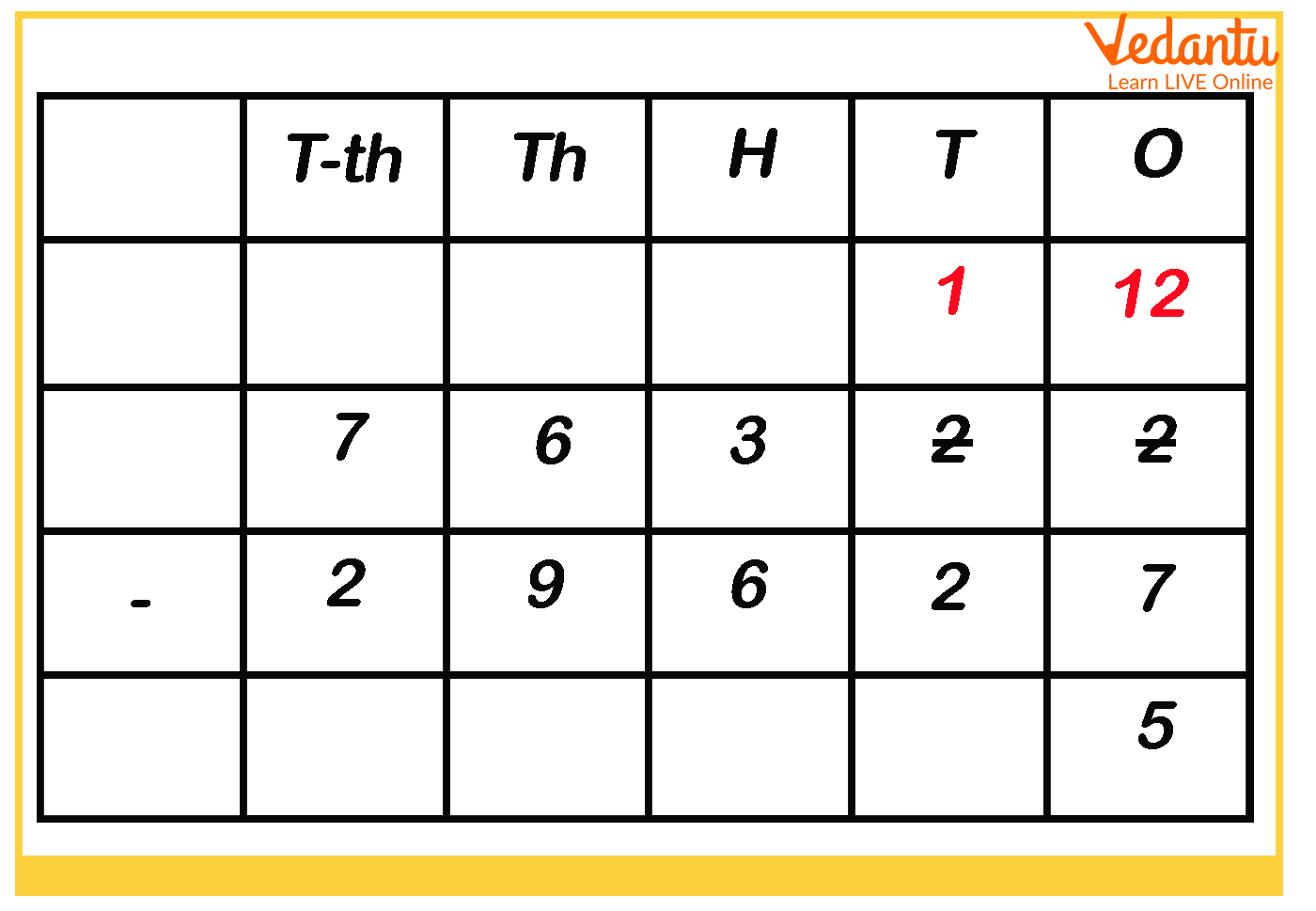Here, we can see that 2 < 7, so 1 tens is borrowed, and 1 ten + 2 ones makes it 12.

Hence, 12 - 7 = 5 ones.

Step 2 - Now, subtract the digits under tens place as shown below: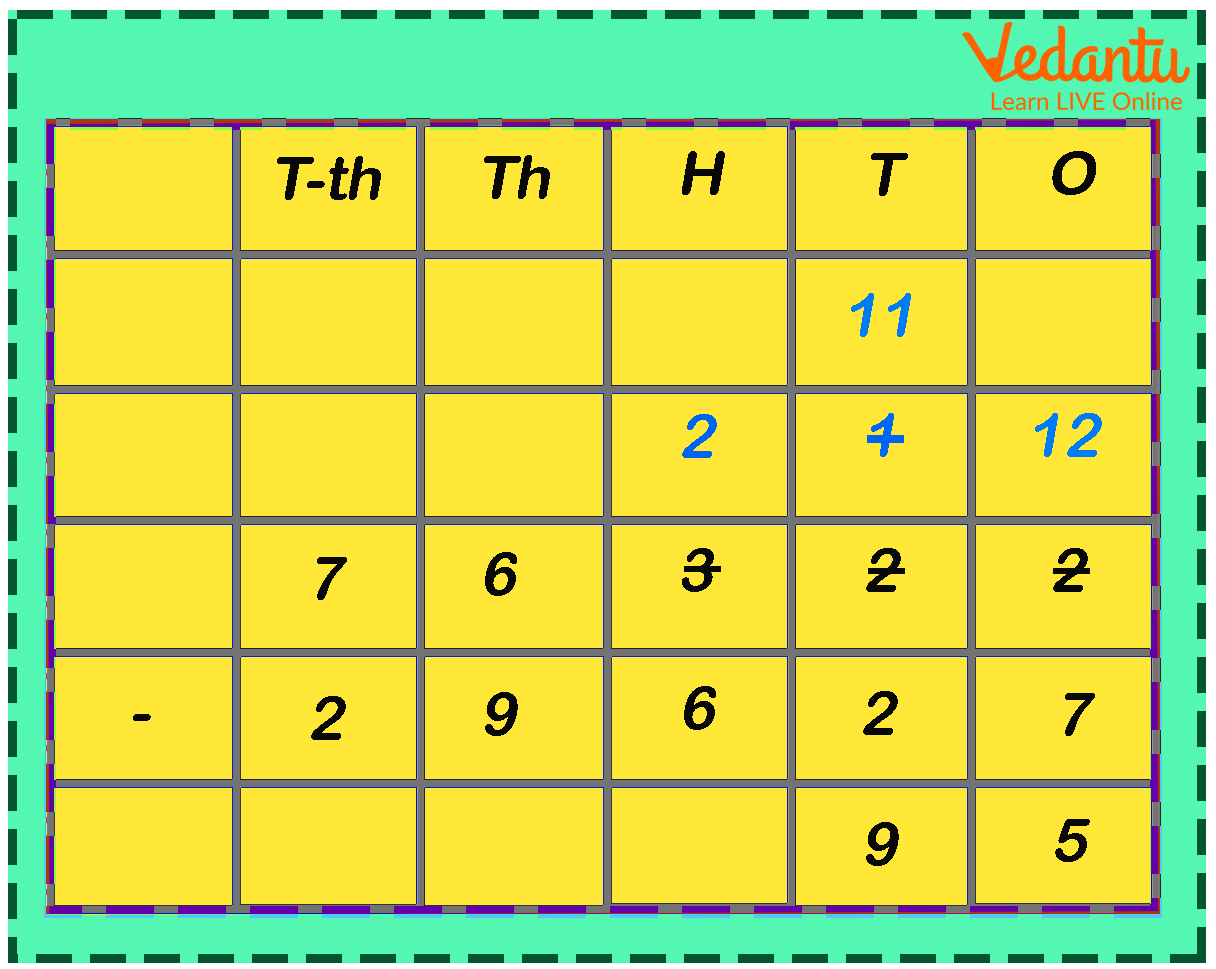Now, 1 ten < 2 tens, so 1 hundred is borrowed and 1 hundred + 1 ten makes it 11 tens.

Hence, 11 tens - 2 tens = 9 tens.

Step 3: Subtract the digits under hundreds place.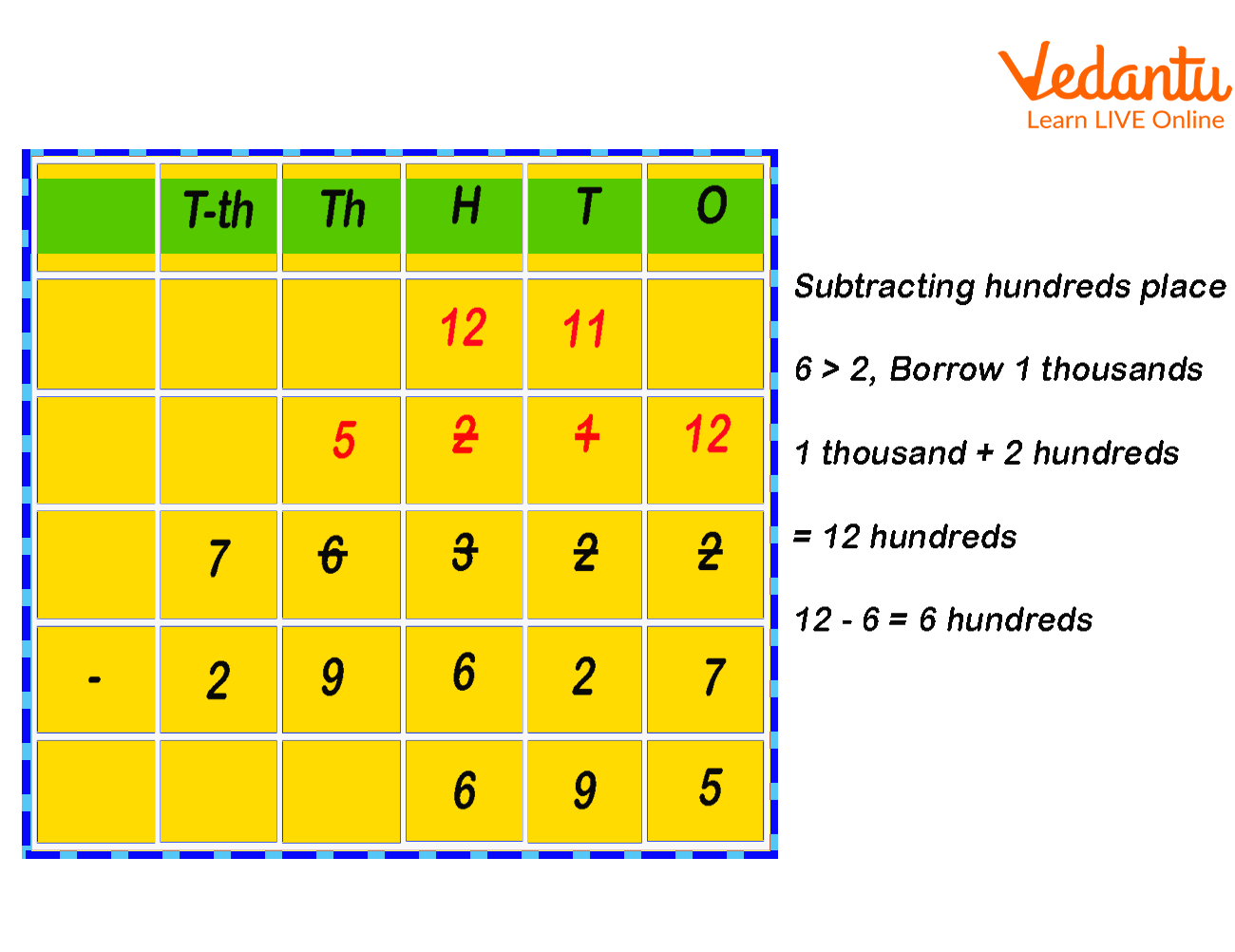Here, 2 hundreds < 6 hundreds, so 1 thousand is borrowed and 1 thousand + 2 hundreds makes it 12 hundred.

Hence, 12 - 6 = 6 hundreds.

Step 4: Subtracting the digits under thousands place.

Here, 5 thousands < 9 thousands, so 1 ten-thousand is borrowed and 1 ten-thousand + 5 thousands makes it 15 thousands.

Hence, 15 - 9 = 6 thousands.

Now, we are left with the digit at ten-thousands place, which is 6 in place of 7, so 6 - 2 = 4.

Hence, our result by subtracting 29627 from 76322, comes out as 76322 − 29627 = 46695.

So, this is how we subtract two three-digit or four-digit numbers by using the concept of subtraction without or with borrowing along with the concept of place value.

## Conclusion

Learning Maths might be troublesome for the students as well as teaching the kids Maths for the tutors or parents, in that case, gradual and baby steps introduction to the child is suggested.

In this article, we have covered the subtraction method of 3 and 4-digit numbers both by carrying and without carrying. Make your child practise the sums with different numbers and give them ample fun workbooks to complete the calculations. And in no time, you will find your child excelling in these Mathematical calculations.

Last updated date: 28th Sep 2023
Total views: 132.6k
Views today: 1.32k

## FAQs on Subtraction Year 3 - Learn Subtraction with Examples

1. Total population of a city is 75555, out of which 37886 are women and the remaining are men. How many men live in a town?

Here, we have the total population of a city as 75555. The number of women is 37886.

Now, to find the number of men, we need to subtract the number of women from the total population, which is 75555 - 37886. So, by using the concept of subtraction with borrowing, we get our answer as 37669.

Hence, the number of men in a city is 37669.

2. Subtract 237412 from 649523.

3 > 1; so, 3 – 2 = 1 Ones

(ii) 2 Tens > 1 tens; so, 2 – 1 = 1 Tens

(iii) 5 Hundreds > 4 hundreds; so, 5 – 4 = 1 Hundred

(iv) 9 Thousands > 7 thousands; so, 9 – 7 = 2 Thousands

(v) 4 Ten-thousands > 3 ten-thousands; so, 4 – 3 = 1 Ten thousands

(vi) 6 Hundred thousands > 2 hundred thousands; so, 6 – 2 = 4 Hundred thousands

Therefore, we get 412111 as answer.